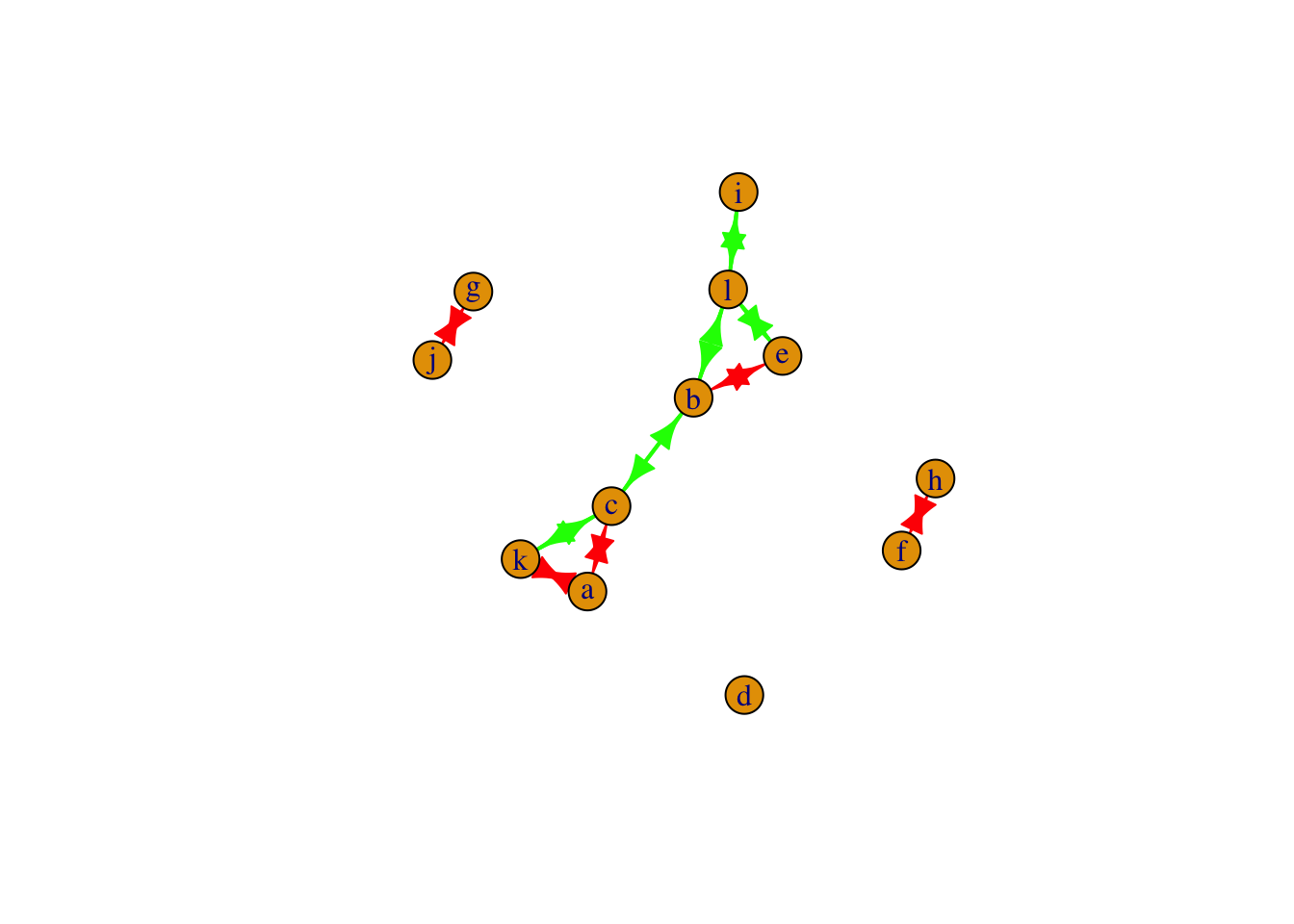## network 版

set.seed(110)
library(network)
# 生成一个3节点网络
net <- network.initialize(3)
# 画出来
plot(net,vertex.cex=10)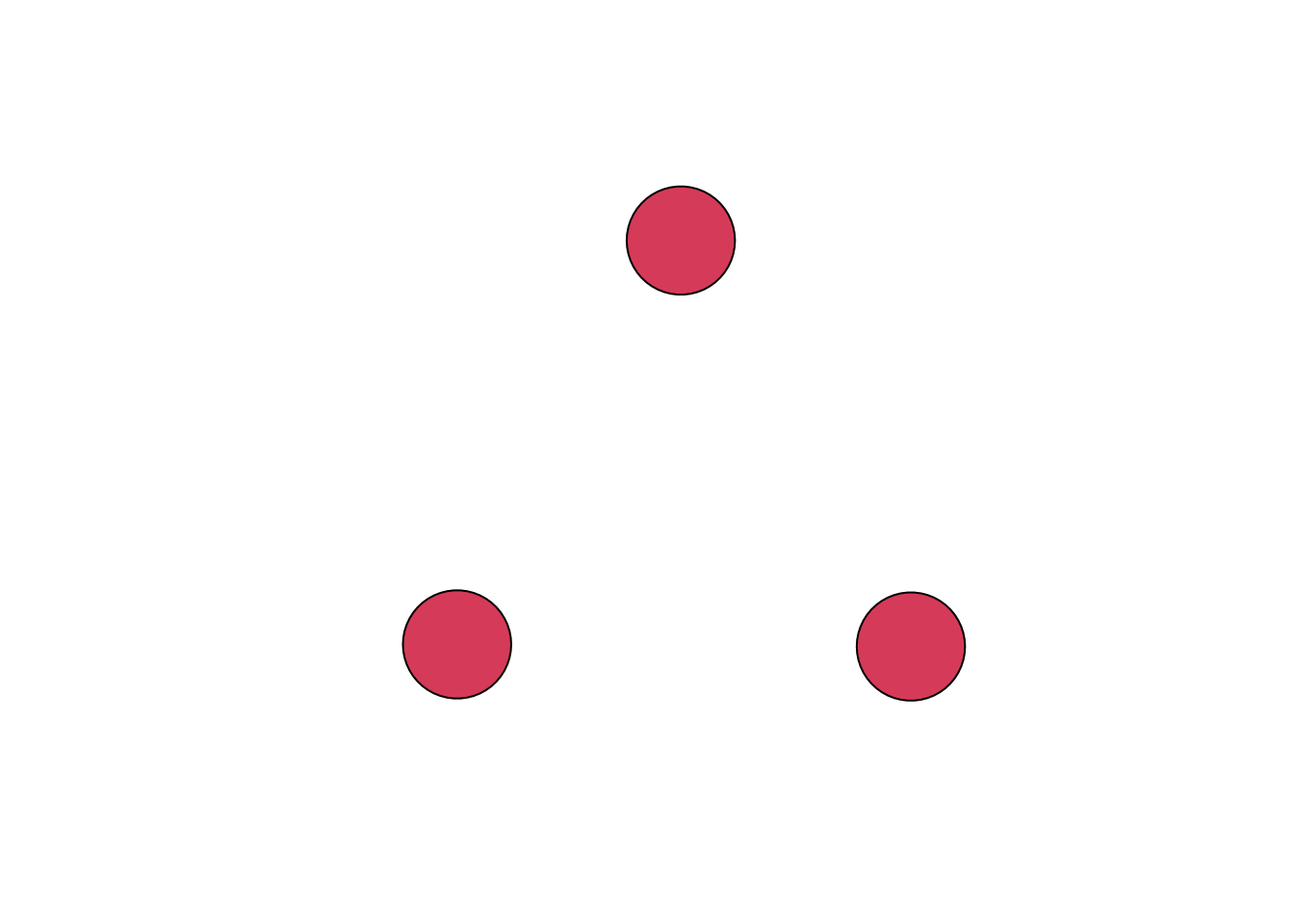# 添加一条边
# 画出来
plot(net,vertex.cex=10, displaylabels=T)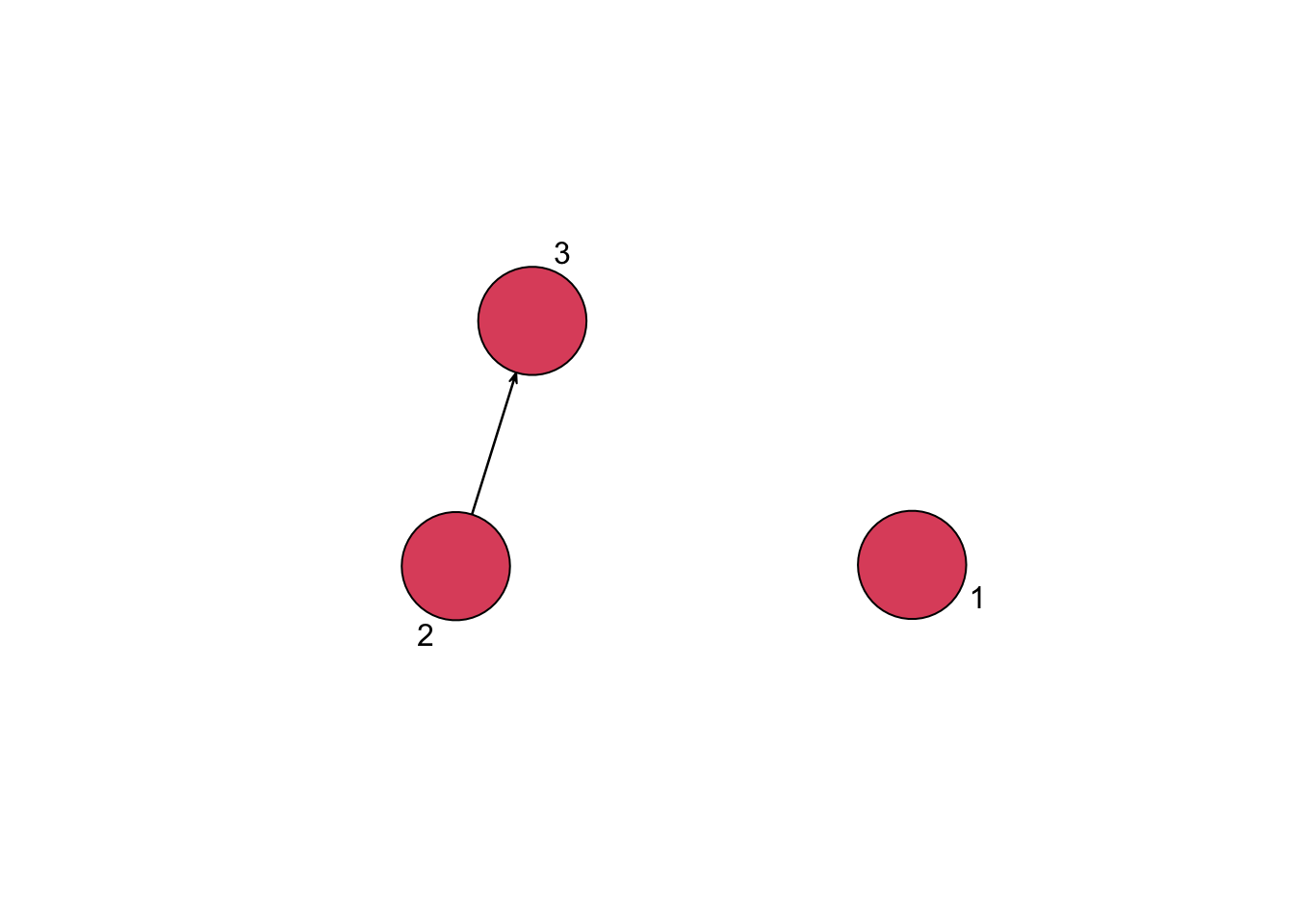# 添加两个点
# 画出来
plot(net,vertex.cex=10, displaylabels=T)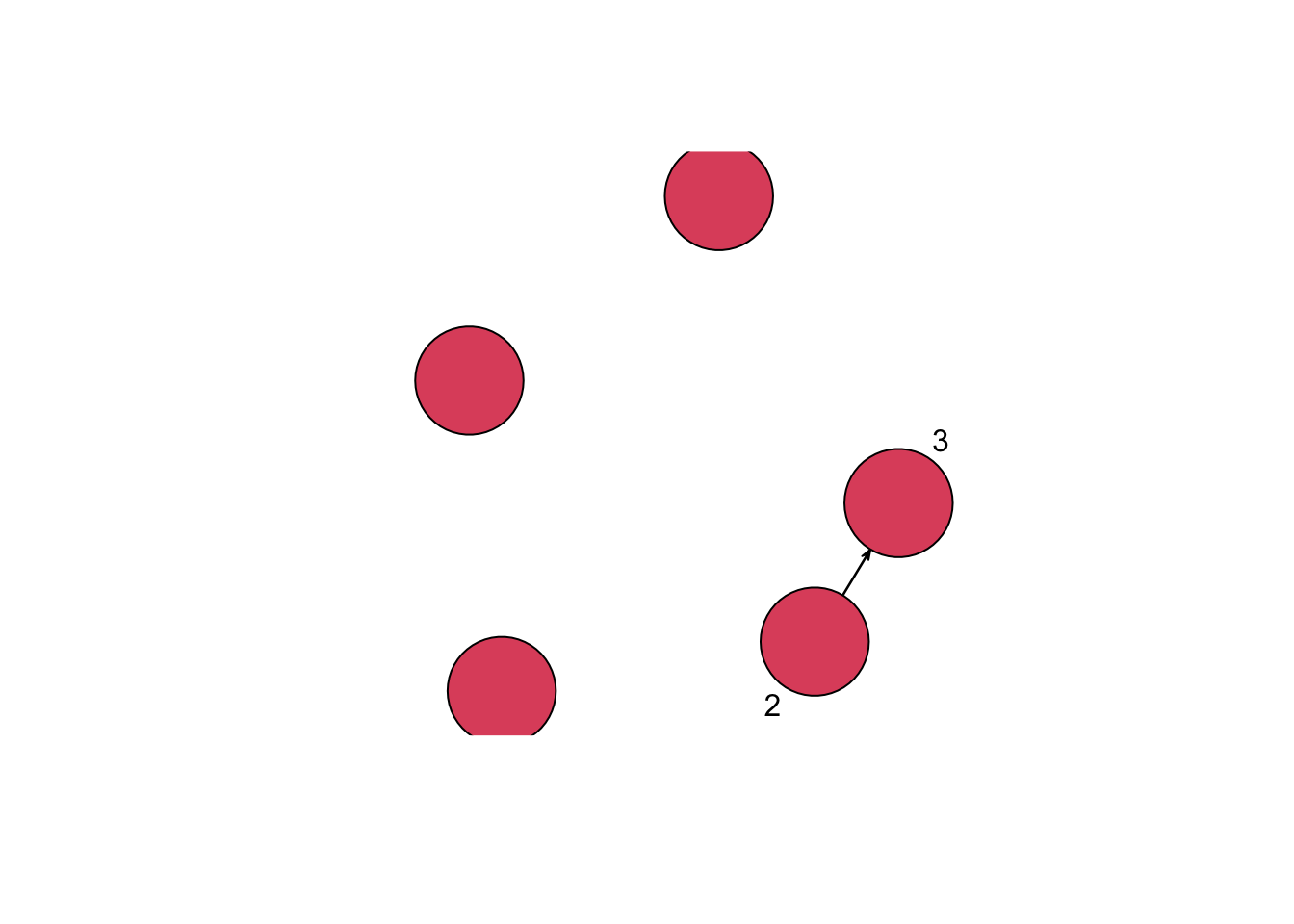# 模拟一个5*12的数据框
df <- matrix(rnorm(60),5)
# 用邻接矩阵直接生成网络
dfcor <- cor(df)
# 去掉低相关性边
dfcor[dfcor<0.5] <- 0
plot(netcor)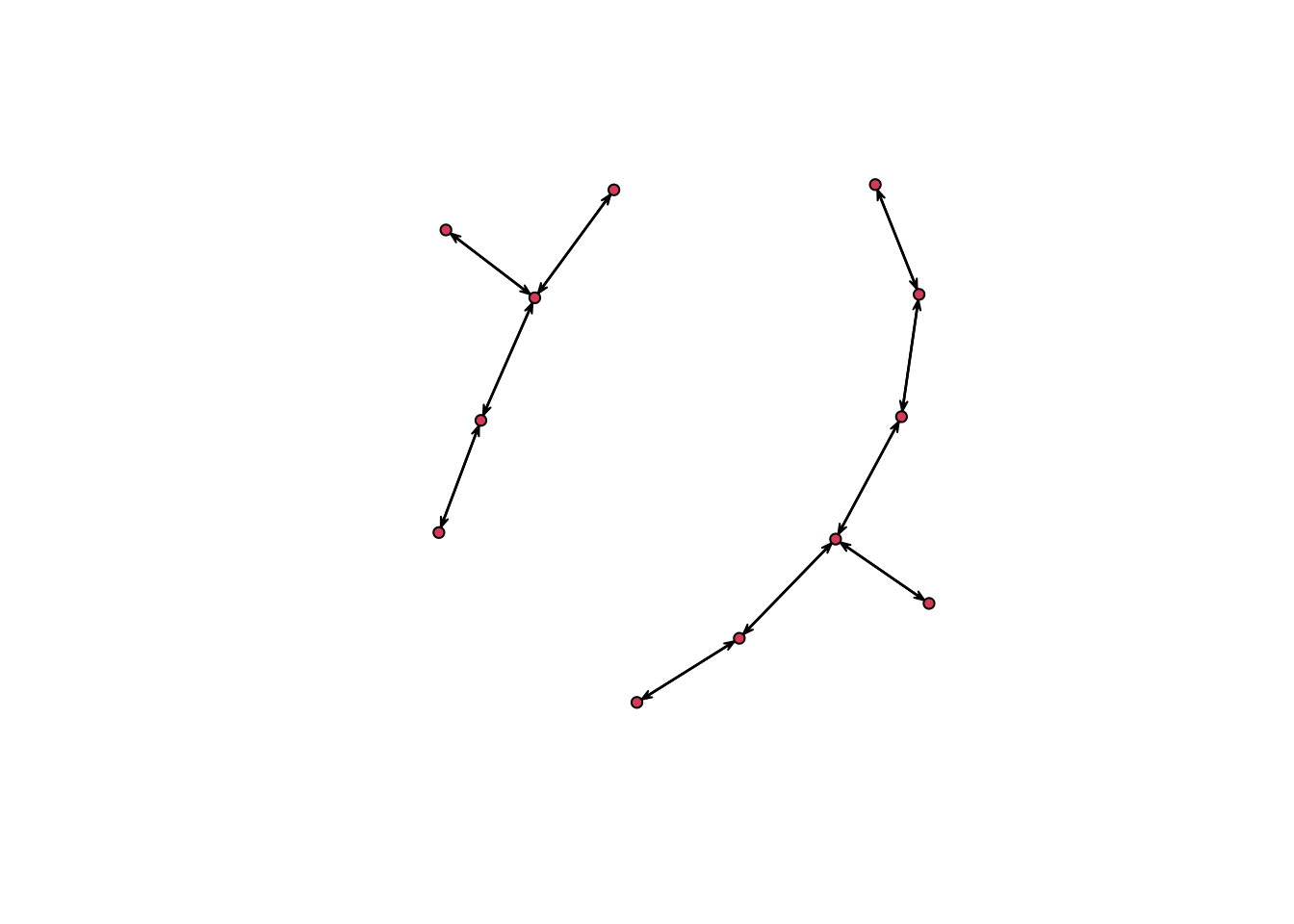# 增加节点/边属性
set.vertex.attribute(netcor, "class", length(netcor$val):1) set.edge.attribute(netcor,"color",length(netcor$mel):1)
# 可视化属性
plot(netcor,vertex.cex=5,vertex.col=get.vertex.attribute(netcor,"class"),edge.col=get.edge.attribute(netcor,'color'))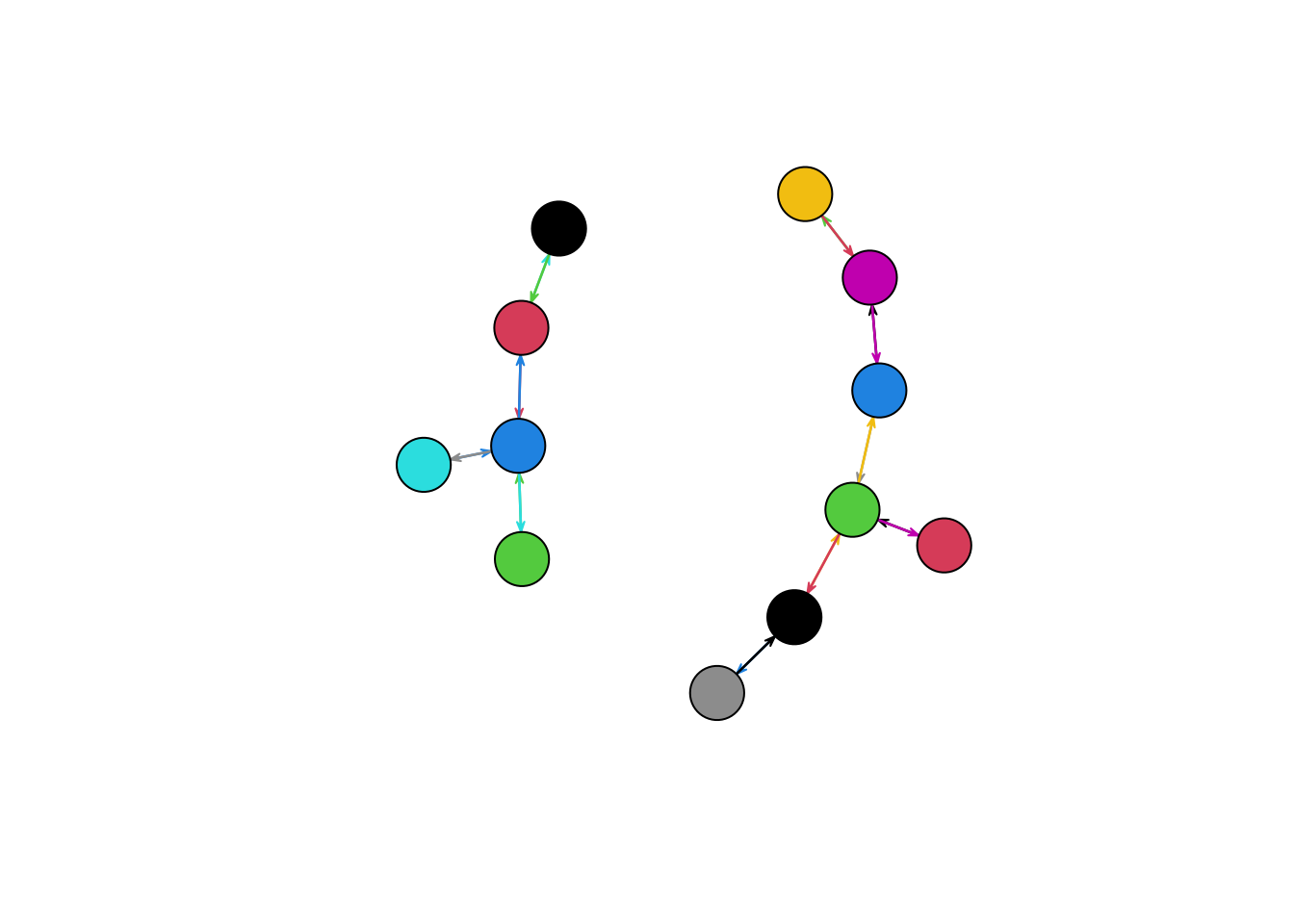## igraph 版

set.seed(110)
library(igraph)
# 生成一个3节点网络
net <- graph.empty(n=3, directed=TRUE)
# 画出来
plot(net)# 添加两条边
new_edges <- c(1,3, 2,3)
# 画出来
plot(net)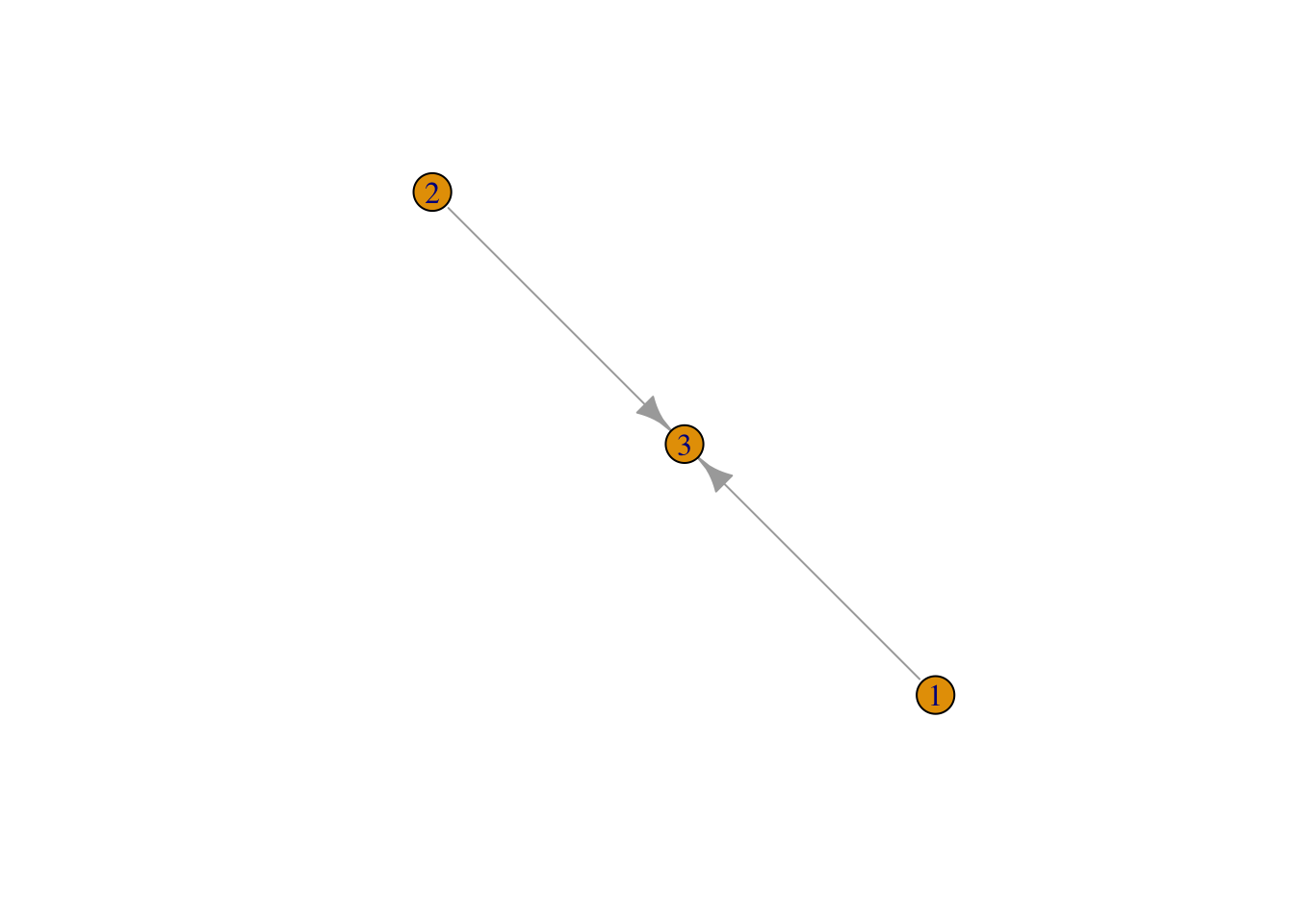# 添加两个点
# 画出来
plot(net)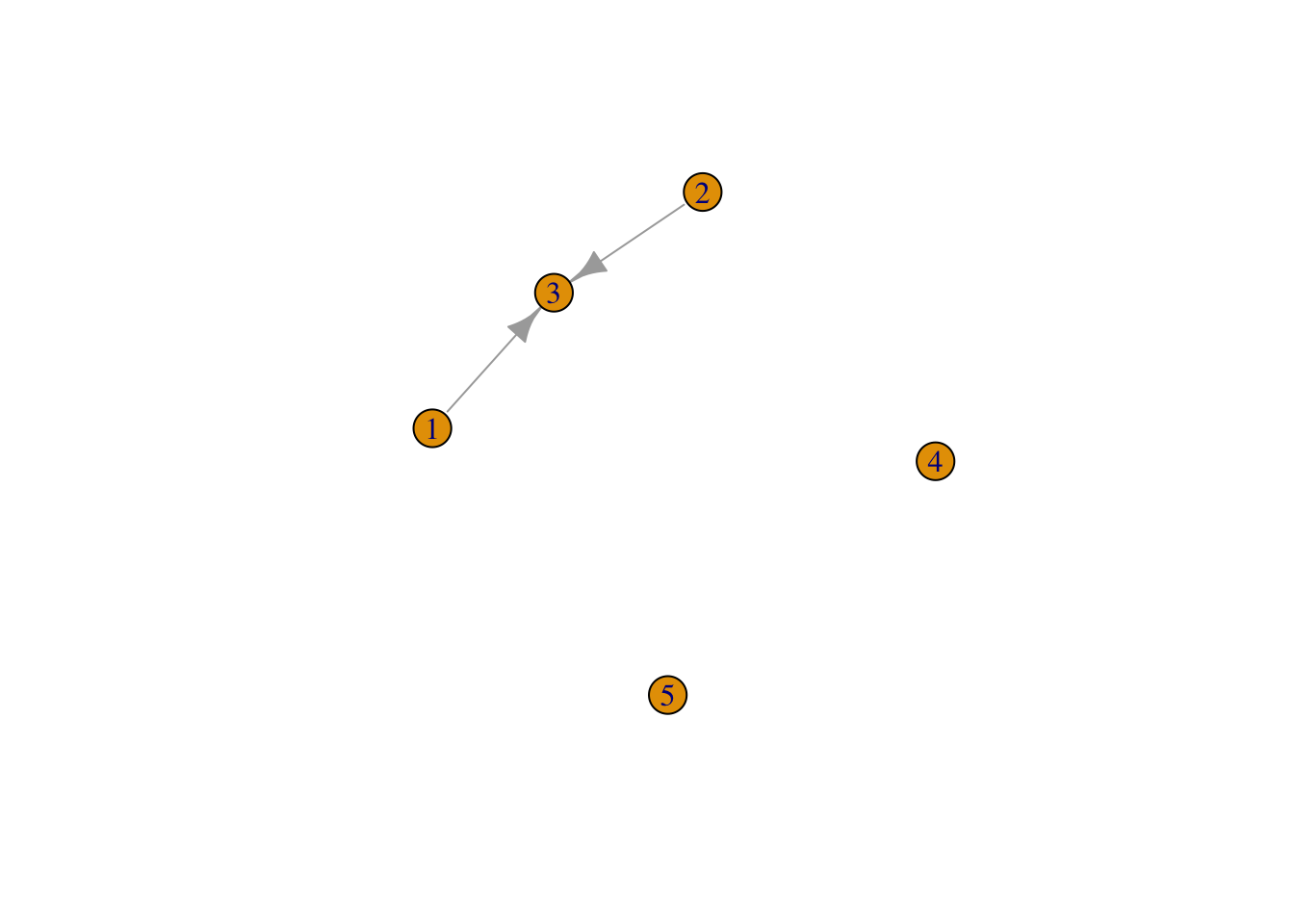# 模拟一个5*12的数据框
df <- matrix(rnorm(60),5)
# 用邻接矩阵直接生成网络
dfcor <- cor(df)
# 去掉低相关性边
dfcor[dfcor<0.5] <- 0
plot(net)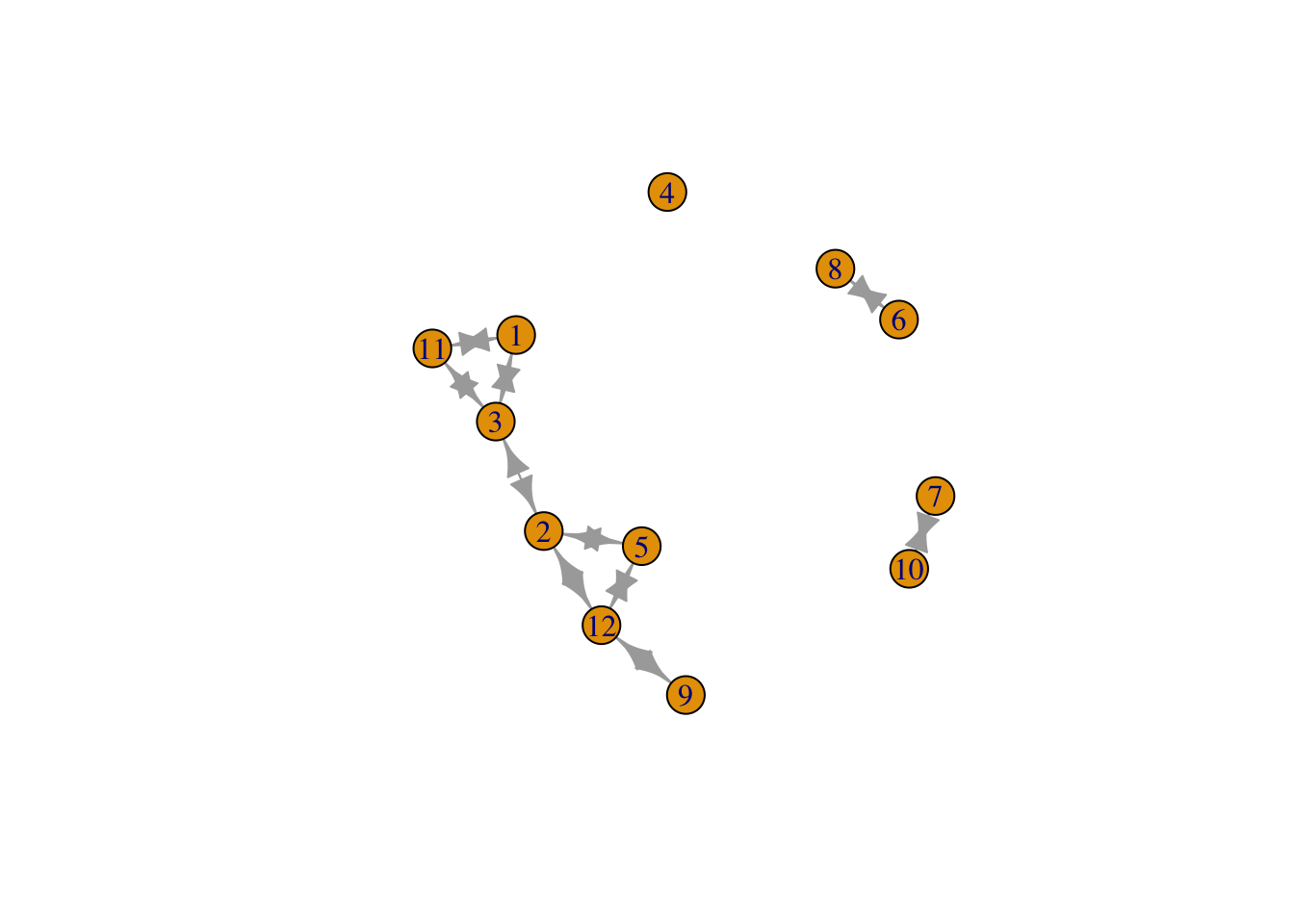# 增加节点/边属性
V(net)$name <- letters[1:vcount(net)] E(net)$color <- "red"
E(net)[ weight < 0.7 ]$width <- 2 E(net)[ weight < 0.7 ]$color <- "green"
# 可视化属性
plot(net)